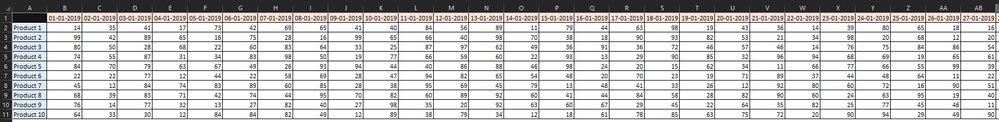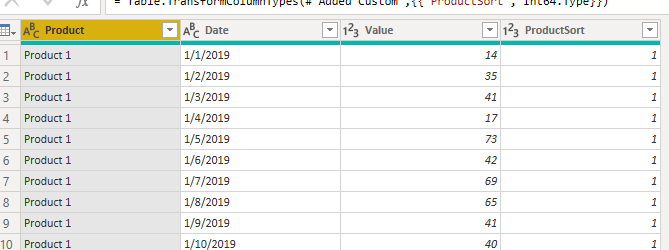cancel
Showing results for
Did you mean:

Fabric is Generally Available. Browse Fabric Presentations. Work towards your Fabric certification with the Cloud Skills Challenge.Frequent Visitor

## Price Index Table Over the Time

Dear All!

We have a table like below:Screenshot UP,

We have several products with their prices over the time since 01-01-2019 so far...

We need a table in Power Bi that show the price indexes on the indexes below:

• 1 Day
• 1 Week
• 1 Month
• 1 Quarter
• 6 Months
• 1 Year
• 2 Years
• 3 Years

e.g. Product 1 has 12% decrease its price in the past 24 hrs,

Product 3 has 44% increased in its price since 6 months ago,

Something like below:Many thanks for the help,

2 ACCEPTED SOLUTIONSCommunity Support

1. You can transpose the table in Power Query Editor and then add a new column to assist [Product] sorting. Like this.2. then please create measures like:

``````1 Day =
VAR _DATE_1 = MAX('Sheet1'[Date])
VAR _DATE_2 = MAX('Sheet1'[Date])-1
VAR _VALUE_1 = CALCULATE(MAX('Sheet1'[Value]),'Sheet1'[Date]= _DATE_1)
VAR _VALUE_2 = CALCULATE(MAX('Sheet1'[Value]),'Sheet1'[Date]=_DATE_2)
VAR _RATE = DIVIDE(_VALUE_1-_VALUE_2,_VALUE_2)
RETURN
_RATE``````
``````1 Week =
VAR _DATE_1 = MAX('Sheet1'[Date])
VAR _DATE_2 = MAX('Sheet1'[Date])-7
VAR _VALUE_1 = CALCULATE(MAX('Sheet1'[Value]),'Sheet1'[Date]= _DATE_1)
VAR _VALUE_2 = CALCULATE(MAX('Sheet1'[Value]),'Sheet1'[Date]=_DATE_2)
VAR _RATE = DIVIDE(_VALUE_1-_VALUE_2,_VALUE_2)
RETURN
_RATE``````
``````1 Month =
VAR _DATE_1 = MAX('Sheet1'[Date])
VAR _DATE_2 = EDATE(MAX('Sheet1'[Date]),-1)
VAR _VALUE_1 = CALCULATE(MAX('Sheet1'[Value]),'Sheet1'[Date]= _DATE_1)
VAR _VALUE_2 = CALCULATE(MAX('Sheet1'[Value]),'Sheet1'[Date]=_DATE_2)
VAR _RATE = DIVIDE(_VALUE_1-_VALUE_2,_VALUE_2)
RETURN
_RATE``````

...

``````3 Year =
VAR _DATE_1 = MAX('Sheet1'[Date])
VAR _DATE_2 = EDATE(MAX('Sheet1'[Date]),-36)
VAR _VALUE_1 = CALCULATE(MAX('Sheet1'[Value]),'Sheet1'[Date]= _DATE_1)
VAR _VALUE_2 = CALCULATE(MAX('Sheet1'[Value]),'Sheet1'[Date]=_DATE_2)
VAR _RATE = DIVIDE(_VALUE_1-_VALUE_2,_VALUE_2)
RETURN
_RATE``````

3. result:Best Regards,
Gao

Community Support Team

If there is any post helps, then please consider Accept it as the solution  to help the other members find it more quickly. If I misunderstand your needs or you still have problems on it, please feel free to let us know. Thanks a lot!

How to get your questions answered quickly -- How to provide sample dataCommunity Support

How about adding a condition to return the Friday date if it is a weekend? Like this:

``````1 Week =
VAR _DATE_1 = MAX('Sheet1'[Date])
VAR _DATE_2 = MAX('Sheet1'[Date])-7
VAR _DETE_3 = SWITCH(TRUE(),WEEKDAY(_DATE_2,2)=6,_DATE_2-1,WEEKDAY(_DATE_2,2)=7,_DATE_2-2,_DATE_2)
VAR _VALUE_1 = CALCULATE(MAX('Sheet1'[Value]),'Sheet1'[Date]= _DATE_1)
VAR _VALUE_2 = CALCULATE(MAX('Sheet1'[Value]),'Sheet1'[Date]=_DETE_3)
VAR _RATE = DIVIDE(_VALUE_1-_VALUE_2,_VALUE_2)
RETURN
_RATE``````

Best Regards,
Gao

Community Support Team

6 REPLIES 6Frequent Visitor

We have an issue here,

We have collected the only business days price over the time,

Meaning, we do not have the prices for Saturdays and Sundays,

So, if the last day or last month or ... would be a weekend day,

The new measures do not return any value 😞Community Support

How about adding a condition to return the Friday date if it is a weekend? Like this:

``````1 Week =
VAR _DATE_1 = MAX('Sheet1'[Date])
VAR _DATE_2 = MAX('Sheet1'[Date])-7
VAR _DETE_3 = SWITCH(TRUE(),WEEKDAY(_DATE_2,2)=6,_DATE_2-1,WEEKDAY(_DATE_2,2)=7,_DATE_2-2,_DATE_2)
VAR _VALUE_1 = CALCULATE(MAX('Sheet1'[Value]),'Sheet1'[Date]= _DATE_1)
VAR _VALUE_2 = CALCULATE(MAX('Sheet1'[Value]),'Sheet1'[Date]=_DETE_3)
VAR _RATE = DIVIDE(_VALUE_1-_VALUE_2,_VALUE_2)
RETURN
_RATE``````

Best Regards,
Gao

Community Support TeamFrequent Visitor

Much appreciated 🙂

That's it!Frequent Visitor

Many thanks for the help 🙂Community Support

1. You can transpose the table in Power Query Editor and then add a new column to assist [Product] sorting. Like this.2. then please create measures like:

``````1 Day =
VAR _DATE_1 = MAX('Sheet1'[Date])
VAR _DATE_2 = MAX('Sheet1'[Date])-1
VAR _VALUE_1 = CALCULATE(MAX('Sheet1'[Value]),'Sheet1'[Date]= _DATE_1)
VAR _VALUE_2 = CALCULATE(MAX('Sheet1'[Value]),'Sheet1'[Date]=_DATE_2)
VAR _RATE = DIVIDE(_VALUE_1-_VALUE_2,_VALUE_2)
RETURN
_RATE``````
``````1 Week =
VAR _DATE_1 = MAX('Sheet1'[Date])
VAR _DATE_2 = MAX('Sheet1'[Date])-7
VAR _VALUE_1 = CALCULATE(MAX('Sheet1'[Value]),'Sheet1'[Date]= _DATE_1)
VAR _VALUE_2 = CALCULATE(MAX('Sheet1'[Value]),'Sheet1'[Date]=_DATE_2)
VAR _RATE = DIVIDE(_VALUE_1-_VALUE_2,_VALUE_2)
RETURN
_RATE``````
``````1 Month =
VAR _DATE_1 = MAX('Sheet1'[Date])
VAR _DATE_2 = EDATE(MAX('Sheet1'[Date]),-1)
VAR _VALUE_1 = CALCULATE(MAX('Sheet1'[Value]),'Sheet1'[Date]= _DATE_1)
VAR _VALUE_2 = CALCULATE(MAX('Sheet1'[Value]),'Sheet1'[Date]=_DATE_2)
VAR _RATE = DIVIDE(_VALUE_1-_VALUE_2,_VALUE_2)
RETURN
_RATE``````

...

``````3 Year =
VAR _DATE_1 = MAX('Sheet1'[Date])
VAR _DATE_2 = EDATE(MAX('Sheet1'[Date]),-36)
VAR _VALUE_1 = CALCULATE(MAX('Sheet1'[Value]),'Sheet1'[Date]= _DATE_1)
VAR _VALUE_2 = CALCULATE(MAX('Sheet1'[Value]),'Sheet1'[Date]=_DATE_2)
VAR _RATE = DIVIDE(_VALUE_1-_VALUE_2,_VALUE_2)
RETURN
_RATE``````

3. result:Best Regards,
Gao

Community Support Team

If there is any post helps, then please consider Accept it as the solution  to help the other members find it more quickly. If I misunderstand your needs or you still have problems on it, please feel free to let us know. Thanks a lot!

How to get your questions answered quickly -- How to provide sample dataSuper User

@ahadtk , You have to create those many change % measures

some examples are here

Time Intelligence, DATESMTD, DATESQTD, DATESYTD, Week On Week, Week Till Date, Custom Period on Period,
Custom Period till date: https://youtu.be/aU2aKbnHuWs&t=145s

Power BI — Year on Year with or Without Time Intelligence
https://medium.com/@amitchandak.1978/power-bi-ytd-questions-time-intelligence-1-5-e3174b39f38a
Power BI — Qtr on Qtr with or Without Time Intelligence
https://medium.com/@amitchandak.1978/power-bi-qtd-questions-time-intelligence-2-5-d842063da839
Power BI — Month on Month with or Without Time Intelligence
https://medium.com/@amitchandak.1978/power-bi-mtd-questions-time-intelligence-3-5-64b0b4a4090e
Power BI — Week on Week and WTD
https://medium.com/@amitchandak.1978/power-bi-wtd-questions-time-intelligence-4-5-98c30fab69d3
https://community.powerbi.com/t5/Community-Blog/Week-Is-Not-So-Weak-WTD-Last-WTD-and-This-Week-vs-La...
Day Intelligence - Last day, last non continous day
https://medium.com/@amitchandak.1978/power-bi-day-intelligence-questions-time-intelligence-5-5-5c324...

Others here

https://medium.com/chandakamit/power-bi-when-i-felt-lazy-and-i-needed-too-many-measures-ed8de20d9f79Announcements#### Power BI Monthly Update - November 2023

Check out the November 2023 Power BI update to learn about new features.#### Fabric Community News unified experience

Read the latest Fabric Community announcements, including updates on Power BI, Synapse, Data Factory and Data Activator.#### The largest Power BI and Fabric virtual conference

130+ sessions, 130+ speakers, Product managers, MVPs, and experts. All about Power BI and Fabric. Attend online or watch the recordings.Top Solution Authors
Top Kudoed Authors
Users online (1,639)